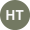# How to solve quadratic equations with Delta Math2 months ago

Quadratic equations are one of the most common types of equations in algebra. They have the form ax^2 + bx + c = 0, where a, b, and c are constants, and x is the unknown variable. Solving quadratic equations can be tricky, but a handy tool can help you: Delta Math.

Delta Math is an online platform that provides interactive math practice and feedback for students and teachers. One of the features of Delta Math is that it can generate and solve quadratic equations for you. Here is how you can use Delta Math to solve quadratic equations:

2. Click on the "Create Assignment" button and choose "Quadratic Equations" from the list of topics.

3. You can customize the difficulty level, the number of questions, the time limit, and other options for your assignment.

4. Click on the "Create Assignment" button again to see a list of quadratic equations Delta Math has generated for you.

5. To solve a quadratic equation, click on it, and you will see a pop-up window with the equation and a hint button.

6. The hint button will show you the steps to solve the equation using the quadratic formula: x = (-b ± √(b^2 - 4ac)) / 2a.

7. You can also use the graphing tool to see the graph of the equation and its roots (the values of x that make the equation equal to zero).

8. To check your answer, enter it in the box below the equation and click on the "Check Answer" button. Delta Math will tell you if your answer is correct or not and give you feedback.

9. You can repeat this process for all the quadratic equations in your assignment and track your progress and score.

Delta Math is a great way to practice and learn how to solve quadratic equations. It can also help you with other math topics, such as linear equations, functions, polynomials, trigonometry, and more. Delta Math is fun, easy, and effective, so give it a try and see how it can improve your math skills.

If you are struggling with a Delta Math assignment, feel free to contact us, and we will help you. Our tutors are always available to assist.

Share post
Top